Consider the following alcohols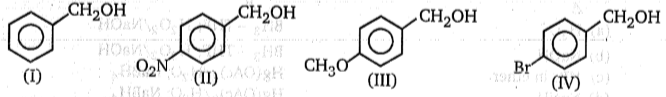The order of decreasing reactivities of these alcohols towards substitution with HBr is :
(a) III > I > IV > II

(b) III > I > II > Ⅳ

(c) I > III > IV > II

(d) I > III > II > IV

High Yielding Test Series + Question Bank - NEET 2020

Difficulty Level:

Predict the product when given compound reacts with LiAlH4 :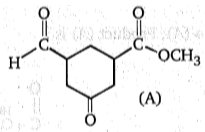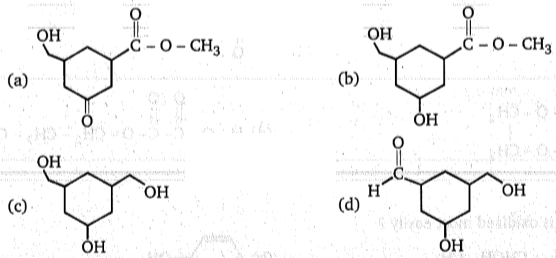High Yielding Test Series + Question Bank - NEET 2020

Difficulty Level: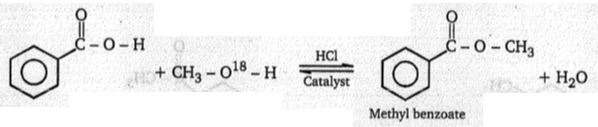The labelled -O18 will be in:
(a) H2O
(b) Methyl benzoate
(c) Both (a) and (b)
(d) Benzoic acid

Concept Questions :-

Alcohols: Preparation, Physical and Chemical Properties
High Yielding Test Series + Question Bank - NEET 2020

Difficulty Level:

$\begin{array}{c}\underset{|}{{\mathrm{CO}}_{2}\mathrm{H}}\\ {\mathrm{CO}}_{2}\mathrm{H}\end{array}+\begin{array}{ccc}\underset{|}{{\mathrm{CH}}_{2}}& -& \stackrel{18}{\mathrm{OH}}\\ {\mathrm{CH}}_{2}& -& \stackrel{18}{\mathrm{OH}}\end{array}\underset{∆}{\overset{{\mathrm{H}}^{+}}{\to }}$ (A); Product (A) is:

(a) $\begin{array}{ccccc}\stackrel{\mathrm{O}}{\stackrel{\parallel }{\underset{|}{\mathrm{C}}}}& -& \mathrm{O}& -& {\mathrm{CH}}_{2}\\ \underset{\mathrm{O}}{\underset{\parallel }{\mathrm{C}}}& -& \mathrm{O}& -& {\mathrm{CH}}_{2}\end{array}$

(b) $\begin{array}{ccccc}\stackrel{\mathrm{O}}{\stackrel{\parallel }{\underset{|}{\mathrm{C}}}}& -& \stackrel{18}{\mathrm{O}}& -& \underset{|}{{\mathrm{CH}}_{2}}\\ \underset{\mathrm{O}}{\underset{\parallel }{\mathrm{C}}}& -& \underset{18}{\mathrm{O}}& -& {\mathrm{CH}}_{2}\end{array}$

(c) $\begin{array}{ccccc}\stackrel{{}^{18}\mathrm{O}}{\stackrel{\parallel }{\underset{|}{\mathrm{C}}}}& -& \mathrm{O}& -& \underset{|}{{\mathrm{CH}}_{2}}\\ \underset{{}^{18}\mathrm{O}}{\underset{\parallel }{\mathrm{C}}}& -& \mathrm{O}& -& {\mathrm{CH}}_{2}\end{array}$

(d) $\begin{array}{ccccccccccccccc}\mathrm{H}& -& \mathrm{O}& -& \stackrel{\mathrm{O}}{\stackrel{\parallel }{\mathrm{C}}}& -& \stackrel{\mathrm{O}}{\stackrel{\parallel }{\mathrm{C}}}& -\mathrm{O}& -& {\mathrm{CH}}_{2}& -& {\mathrm{CH}}_{2}& -& \mathrm{OH}& \end{array}$

Concept Questions :-

Alcohols: Preparation, Physical and Chemical Properties
High Yielding Test Series + Question Bank - NEET 2020

Difficulty Level:

Which is oxidized most easily ?

(a)CH3-CHOH-CH3

(b)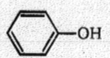(c)CH3-CH2-O-CH2-CH3

(d)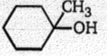Concept Questions :-

Alcohols: Preparation, Physical and Chemical Properties
High Yielding Test Series + Question Bank - NEET 2020

Difficulty Level: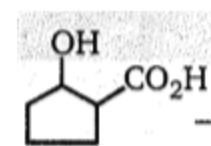$\underset{∆}{\overset{{\mathrm{K}}_{2}{\mathrm{Cr}}_{2}{\mathrm{O}}_{7}}{\to }}$(P); Product (P) is:

(a)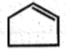(b)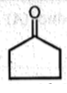(c)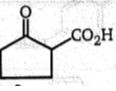(d)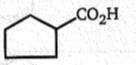Concept Questions :-

Alcohols: Preparation, Physical and Chemical Properties
High Yielding Test Series + Question Bank - NEET 2020

Difficulty Level:

Which of the following react with HBr at faster rate?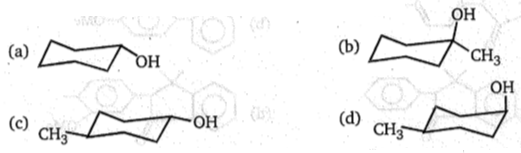Concept Questions :-

Alcohols: Preparation, Physical and Chemical Properties
High Yielding Test Series + Question Bank - NEET 2020

Difficulty Level: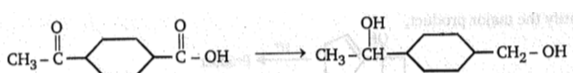Above conversion can be done by:

1. NaBH4

2. LiAlH4

3. PCC

4. KMnO4

Concept Questions :-

Alcohols: Preparation, Physical and Chemical Properties
High Yielding Test Series + Question Bank - NEET 2020

Difficulty Level: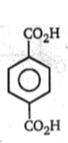$\underset{{\mathrm{H}}_{2}{\mathrm{SO}}_{4}∆}{\overset{2{\mathrm{CH}}_{3}\mathrm{OH}}{\to }}$(A); Product (A) is:

(a)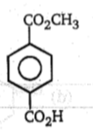(b)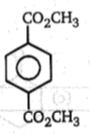(c)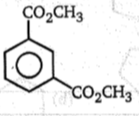(d)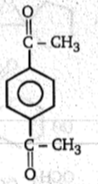Concept Questions :-

Alcohols: Preparation, Physical and Chemical Properties
High Yielding Test Series + Question Bank - NEET 2020

Difficulty Level:

Identify the major product,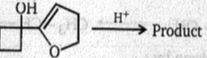(a)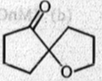(b)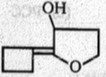(c)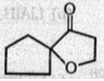(d)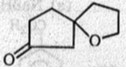Concept Questions :-

Alcohols: Preparation, Physical and Chemical Properties